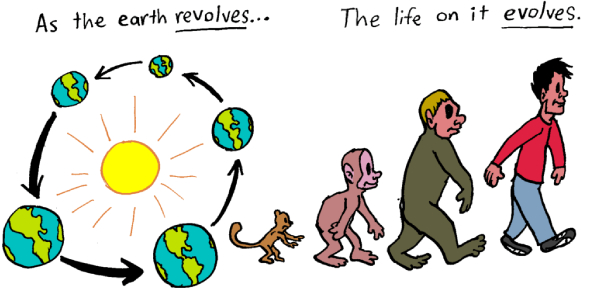Science Vocabulary Basic Quiz!

29 Questions | Total Attempts: 76Settings.

• 1.
Element
• A.

Pure substance

• B.

HCL

• 2.
Compound
• A.

Composed of two or more elements

• B.

Composed of only one element

• 3.
Composed means
• A.

Most abundant

• B.

• 4.
Which are substances?
• A.

Milk, water, air, gold

• B.

Carbon dioxide, hydrogen, carbon, nitrogen

• C.

All of the above

• 5.
Which is an example of chemical symbols?
• A.

Water, aluminum, neon

• B.

H20, Al, Ne

• 6.
• A.

It is a compound composed of 3 different elements

• B.

It is a pure substance

• C.

Its chemical symbol is H2O

• 7.
Abundant means...
• A.

Rare or the least

• B.

Alot or the most

• 8.
Which is a sphere on Earth?
• A.

Universe

• B.

Lithosphere

• 9.
Inferred means?
• A.

Fact

• B.

• 10.
Another word for atoms:
• A.

Sources

• B.

Forms

• C.

Particles

• 11.
A mixture is:
• A.

The same as a compound

• B.

Elements or compounds that are not chemicaly combined

• 12.
A wind generator uses ____________
• A.

Chemical energy from oil

• B.

Mechanical energy from water

• C.

Mechanical energy from wind

• 13.
A gasoline engine uses:
• A.

Nuclear energy from uranium

• B.

Geothermal energy

• C.

Chemical energy from a nonrenewable source

• 14.
Biomass is
• A.

Renewable because you can grow more trees, grass, and crops

• B.

Nonrenewable because it cannot be replaced

• 15.
A solar panel works because of:
• A.

Mechanical energy

• B.

• 16.
Which is not a fossil fuel?
• A.

Coal

• B.

Oil

• C.

Uranium

• 17.
Hydroelectricity
• A.

Is using mechanical energy from wind to generate electricity

• B.

Involves a dam, and flowing water

• 18.
A moving turbine has:
• A.

Chemical energy

• B.

Mechanical energy

• 19.
Conserve means
• A.

To waste

• B.

To save

• 20.
Being energy efficient means?
• A.

Using energy poorly

• B.

Using energy wisely

• 21.
A thermostat
• A.

Controls the amount of natural lighting

• B.

Controls the temperature in a room

• 22.
When heat is transferred from particle to particle in a solid it is called
• A.

Convection

• B.

Conduction

• 23.
Air circulating (heat rises, cold sinks)
• A.

Convection

• B.

Conduction

• 24.
Which is the best conductor of heat?
• A.

Wood

• B.

Metal

• 25.
Thermal energy moves from ________________ until both substances have the same temperature.
• A.

Cooler to warmer

• B.

Warmer to cooler

Related TopicsBack to top